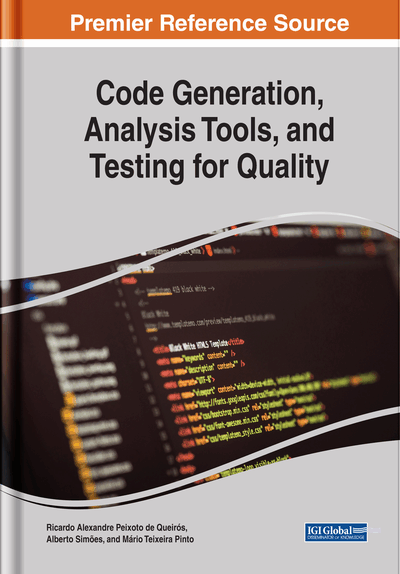Alberto Simões (Polytechnic Institute of Cávado and Ave, Portugal) and Rui Miguel da Costa Meira (Polytechnic Institute of Cávado and Ave, Portugal)
DOI: 10.4018/978-1-5225-7455-2.ch008

## Abstract

This chapter describes an approach for the implementation of embedded domain-specific languages by using operator overloads and the creation of abstract syntax trees in run-time. Using the host language parser, an AST is created stating the structure of the DSL expression that is later analyzed, simplified, and optimized before the evaluation step. For the illustration of this process, the chapter proposes a domain-specific language for a basic linear algebra system dealing with matrices algebra and its optimization.
Chapter Preview
Top

## Motivation

The motivation for the approach here described is the construction of a basic Perl framework to act like TensorFlow, for simple neural networks, and easy to understand and edit by the community. While the module is under work and at a reasonable distance from being really useful, its development arose some problems that lead to the architecture here described.

The implementation of a neural network deals mainly with Basic Linear Algebra (BLA) operations. Given the requirement of fast implementations, these operations were obtained through a Basic Linear Algebra Subprograms (BLAS) library. The more relevant methods were defined: matrix sum and subtraction, element-wise operators (sum, multiplication, exponentiation, etc), matrices inner product, matrix transposition, matrix inverse, sigmoid and tanh, and some other operations.

## Complete Chapter List

Search this Book:
Reset